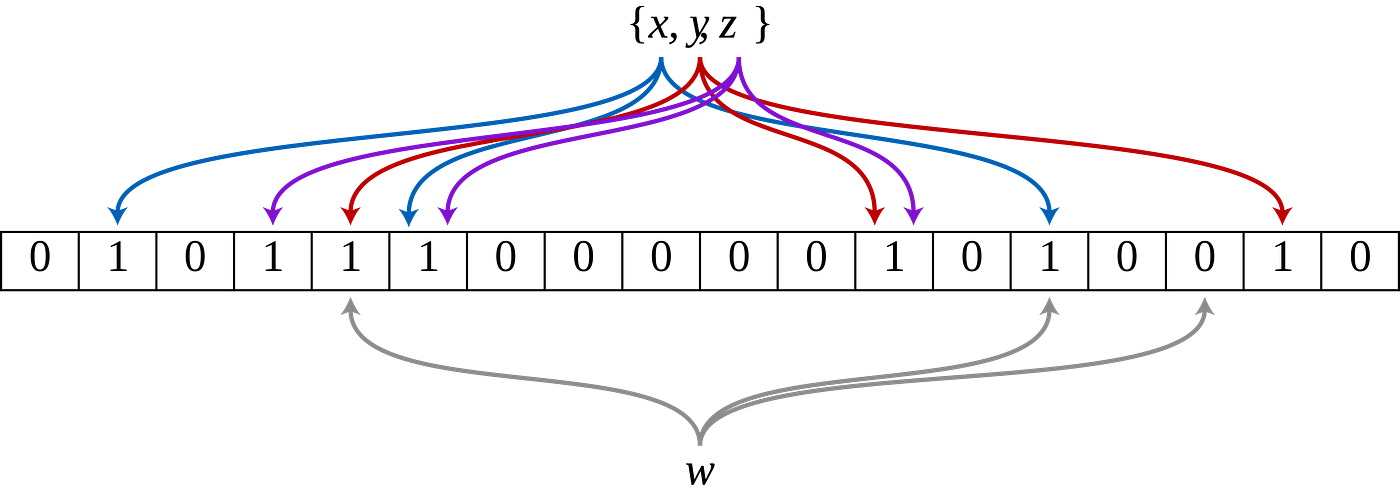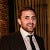# What is a Bloom Filter?

This article is about a data structure called a Bloom Filter. I was asked to write this as part of the M269 module for my Open University degree course in 2018. I thought I would share this here as it has some applications in network routing and may be interesting to some.

A brief explanation of Bloom Filters

A bloom filter is a probabilistic data structure developed by Burton Howard Bloom in 1970. A Bloom Filter is a variant of the Hash Table data structure and is used to test whether an element is a member of a set in a fast and memory efficient way. Bloom filters are used for searching for members in a set in a similar way to the Hash Table data structure. Bloom filters however take up less memory space.

A good application for bloom filters is in the space of network routers; in which fast lookup of IP addresses is essential, and where memory space is at a premium. IP addresses are stored in memory on network routers; and a bloom filter could be used to store a list of blocked IP addresses which is then used by the router to look up whether the incoming or outgoing IP address is contained in the set of blocked IP addresses.Figure 1 — An example of a bloom filter

To implement a bloom filter, we first start with an array of n bits which are all initially set to 0. We then need a collection of independent hash functions which we will call k. The hash functions take in a value and they generate a number i from this value making sure that i < n.

When we call the insert operation to add a new element called x to the set, we will first apply each of the hash functions to the new element x generating an integer value which we will then use to set the position in the bit array created above to a value of 1. Below is a concrete example in Python of how the hash function generated the indices for a bit array of size 11.

`def h(x): return int(“”.join([v for i, v in enumerate(bin(x)[2:]) if i % 2 == 0]), 2) % 11def g(x):  return int(“”.join([v for i, v in enumerate(bin(x)[2:]) if i % 2 == 1]), 2) % 11`

As an example if we try to add the value 25 to our bloom filter we would get the indices h(25) = 5 and g(25) = 2 generated from our hash functions. We can then set the values of the positions 5 and 2 in our bit array to the value 1. This means if we had an bit array of size 11 initialized to 00000000000. When the value 25 is inserted the bit array would become 00100100000.

When we want to add new elements or to lookup members of the bloom filter, we simply calculate the array indices for the new element and check the values of the positions of the new array. If one of the values is 0 then we know that the value we are looking up is not part of the set already. As an example, suppose we try to add the value 118 to the set. We generate the indices from the hash functions as 5 and 3. If we look at the bit array and find that array = 1 but array = 0 then we can know that 118 is not already in the set because array has not been set to 1.

The bloom filter however does have a drawback in that it is susceptible to false positives in which the indices generated from the hash functions and the subsequent lookup into the bit array returns values of 1 for every lookup. Wrongly suggesting that the new value has been inserted when it hasn’t.

The time taken to lookup or add an item to a bloom filter is dependent on the constant k which is the number of hash functions used in the algorithm. Therefore, for insert and search operations the complexity of a these operations on a bloom filter would be O(k).

In summary there are pros and cons of using a Bloom Filter for searching, with the main pro for using a bloom filter being the memory space efficiency discussed above. The main drawback of a bloom filter is in its probabilistic nature of returning a result. It’s probabilistic nature means that there is a small chance that result of searching could return a false positive. What we mean by this is that if we search for an item in the bloom filter, we will get a result that tells us either that the item is definitely not in the set or that the item is possibly in the set. There is a small chance that the search will tell us that an item is in the set even when the item has not previously been added to the set.

References

Srivastav, Prakhar. (2014) Bloom Filters for Dummies [Online]. Available at https://prakhar.me/articles/bloom-filters-for-dummies/

Stanford Algorithms (2017) Bloom Filters The Basics [Online]. Available at https://www.youtube.com/watch?v=zYlxP7F3Z3c

Wikipedia (2018) Bloom filter [Online]. Available at https://en.wikipedia.org/wiki/Bloom_filter

--

--## Lee Dale

I am a lead software developer/cloud architect who has been designing and building software solutions professionally for the last twenty years.